Maths-
General
Easy

Question

# If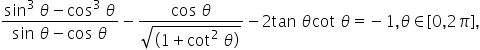,then

##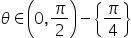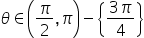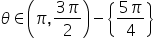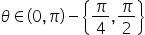Hint:

## The correct answer is:### Here , we have to find where θ lies in this equation.Firstly, we have givenWe know that,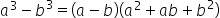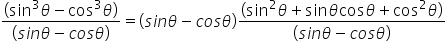= 1 + 2 sin θ cos θ So, we can write, in eq (1)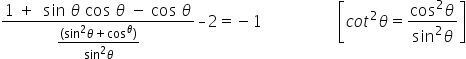sin θ cos θ - |sin θ| cos θ = 0cos θ ( sin θ - | sin θ| ) = 0 hence, sin θ = | sin θ |which is always true for sinx ≥ 0 otherwise it is true for x=0,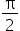,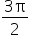,2πTherefore, since we also need x≠,for tanx and x≠0,2π for cotx all the solutions are given by x∈(0,π) with x≠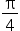and x≠.The correct answer is θ ϵ (0 ,π)- {,}

In this question, we have to find the where is θ lies. Here, always true for sinx ≥ 0 otherwise it is true for x=0,,,2π and since we also need x≠,for tanx and x≠0,2π for cotx all the solutions.#### With Turito Foundation.#### Get an Expert Advice From Turito.